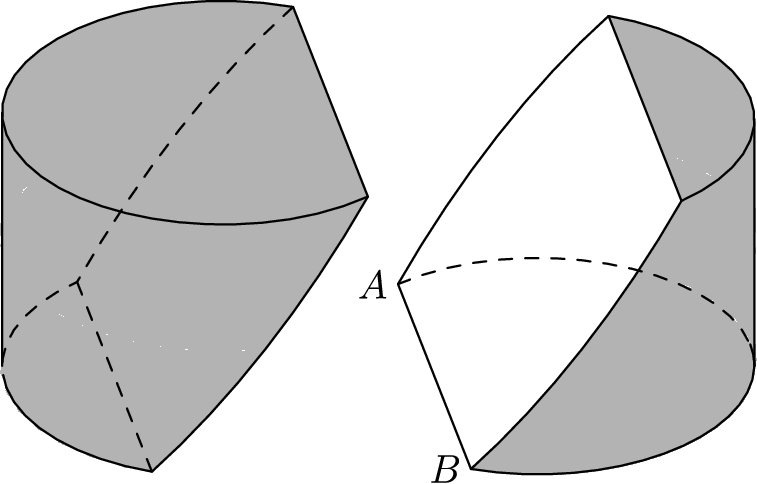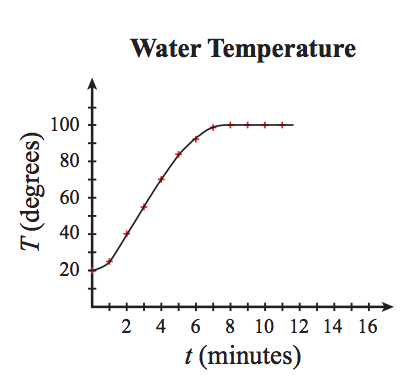#### Methods

###### back to index

Let $n$ be a positive integer, prove that in this series $$\Big\lfloor{\frac{n}{1}}\Big\rfloor, \Big\lfloor{\frac{n}{2}}\Big\rfloor, \Big\lfloor{\frac{n}{3}}\Big\rfloor \cdots \Big\lfloor{\frac{n}{n}}\Big\rfloor$$, there are less than $2\sqrt{n}$ integers distinct.

Solve in integers the equation $$x^2+xy+y^2 = \left(\frac{x+y}{3}+1\right)^3.$$

Quadrilateral $APBQ$ is inscribed in circle $\omega$ with $angle P = \angle Q = 90^{\circ}$ and $AP = AQ < BP$. Let $X$ be a variable point on segment $\overline{PQ}$. Line $AX$ meets $\omega$ again at $S$ (other than $A$). Point $T$ lies on arc $AQB$ of $\omega$ such that $\overline{XT}$ is perpendicular to $\overline{AX}$. Let $M$ denote the midpoint of chord $\overline{ST}$. As $X$ varies on segment $\overline{PQ}$, show that $M$ moves along a circle.

For each integer $n \ge 2$, let $A(n)$ be the area of the region in the coordinate plane defined by the inequalities $1\le x \le n$ and $0\le y \le x \left\lfloor \sqrt x \right\rfloor$, where $\left\lfloor \sqrt x \right\rfloor$ is the greatest integer not exceeding $\sqrt x$. Find the number of values of $n$ with $2\le n \le 1000$ for which $A(n)$ is an integer.

A block of wood has the shape of a right circular cylinder with radius $6$ and height $8$, and its entire surface has been painted blue. Points $A$ and $B$ are chosen on the edge of one of the circular faces of the cylinder so that $\overset\frown{AB}$ on that face measures $120^\text{o}$. The block is then sliced in half along the plane that passes through point $A$, point $B$, and the center of the cylinder, revealing a flat, unpainted face on each half. The area of one of these unpainted faces is $a\cdot\pi + b\sqrt{c}$, where $a$, $b$, and $c$ are integers and $c$ is not divisible by the square of any prime. Find $a+b+c$.Let $m$ be the least positive integer divisible by $17$ whose digits sum is $17$. Find $m$.

How many ordered pairs of positive integers $(x, y)$ can satisfy the equation $x^2 + y^2 = x^3$?

Solve in positive integers $x^2 - 4xy + 5y^2 = 169$.

Let $b$ and $c$ be two positive integers, and $a$ be a prime number. If $a^2 + b^2 = c^2$, prove $a < b$ and $b+1=c$.

How many ordered pairs of positive integers $(a, b, c)$ that can satisfy $$\left\{\begin{array}{ll}ab + bc &= 44\\ ac + bc &=23\end{array}\right.$$

Solve in integers the equation $2(x+y)=xy+7$.

Solve in integers the question $x+y=x^2 -xy + y^2$.

Find the ordered pair of positive integers $(x, y)$ with the largest possible $y$ such that $\frac{1}{x} - \frac{1}{y}=\frac{1}{12}$ holds.

How many ordered pairs of integers $(x, y)$ satisfy $0 < x < y$ and $\sqrt{1984} = \sqrt{x} + \sqrt{y}$?

Find the number of positive integers solutions to $x^2 - y^2 = 105$.

Find any positive integer solution to $x^2 - 51y^2 = 1$.

Solve in positive integers $\frac{1}{x} + \frac{1}{y} + \frac{1}{z} = \frac{4}{5}$

Find all ordered pairs of integers $(x, y)$ that satisfy the equation $$\sqrt{y-\frac{1}{5}} + \sqrt{x-\frac{1}{5}} = \sqrt{5}$$

Solve in integer: $36((xy+1)z+x)=475(yz+1)$

The number $2^{29}$ is a nine-digit number whose digits are all distinct. Which digit of $0$ to $9$ does not appear?

Integers $x$ and $y$ with $x>y>0$ satisfy $x+y+xy=80$. What is $x$?

This graph shows the water temperature T degrees at time t minutes for a pot of water placed on a stove and heated to 100 degrees. On average, how many degrees did the temperature increase each minute during the first $8$ minutes?A circle of radius 2 is centered at $A$. An equilateral triangle with side 4 has a vertex at $A$. What is the difference between the area of the region that lies inside the circle but outside the triangle and the area of the region that lies inside the triangle but outside the circle?

Three congruent isosceles triangles are constructed with their bases on the sides of an equilateral triangle of side length $1$. The sum of the areas of the three isosceles triangles is the same as the area of the equilateral triangle. What is the length of one of the two congruent sides of one of the isosceles triangles?

A rectangle of perimeter 22 cm is inscribed in a circle of area $16\pi$ $cm^2$. What is the area of the rectangle? Express your answer as a decimal to the nearest tenth.